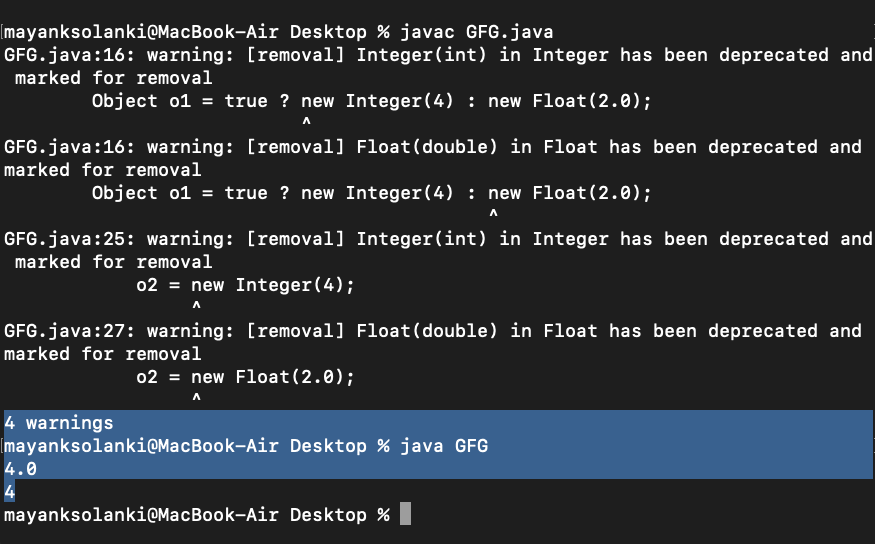Open in App
Not now

# Java Numeric Promotion in Conditional Expression

• Difficulty Level : Medium
• Last Updated : 16 Dec, 2021

With recurrence use of if-else condition in programming which is tend to occur no matter how much we optimize our code. So taking this factor into consideration, conditional operator was introduced making our job of writing code easier as it do have a specific syntax in accordance to which it does check the condition passed to it. The conditional operator ? : uses the boolean value of one expression to decide which of two other expressions should be evaluated. Let us do have an illustration to get used to with its syntax.

Illustration:

```Object obj ;
if (true) {
obj = new Integer(4) ;
} else {
obj = new Float(2.0);
}```

So, with the usage of conditional operator we expect the expression whose syntax is as follows:

`Object obj = true ? new Integer(4) : new Float(2.0));`

Note: But the result of running the code gives an unexpected result.

Example:

## Java

 `// Java Program to Demonstrate``// Replacing of Conditional Operator``// with If-else and viceversa` `// Importing required classes``import` `java.io.*;` `// Main class``class` `GFG {` `    ``// Main driver method``    ``public` `static` `void` `main (String[] args) {` `        ``// Expression 1 (using ?: )``        ``// Automatic promotion in conditional expression``        ``Object o1 = ``true` `? ``new` `Integer(``4``) : ``new` `Float(``2.0``);` `        ``// Printing the output using conditional operator``        ``System.out.println(o1);` `        ``// Expression 2 (Using if-else)``        ``// No promotion in if else statement``        ``Object o2;``        ``if` `(``true``)``            ``o2 = ``new` `Integer(``4``);``        ``else``            ``o2 = ``new` `Float(``2.0``);` `        ``// Printing the output using if-else statement``        ``System.out.println(o2);``    ``}``}`

Output:According to Java Language Specification Section 15.25, the conditional operator will implement numeric type promotion if there are two different types as 2nd and 3rd operand.  The rules of conversion are defined at Binary Numeric Promotion. Therefore, according to the rules given, If either operand is of type double, the other is converted to double and hence 4 becomes 4.0. Whereas, the, if/else construct, does not perform numeric promotion and hence behaves as expected.